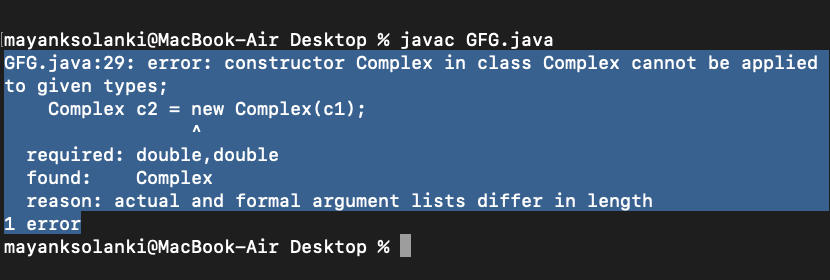# Copy Constructor in Java

• Difficulty Level : Easy
• Last Updated : 16 Jun, 2022

Like C++, Java also supports a copy constructor. But, unlike C++, Java doesn’t create a default copy constructor if you don’t write your own. A prerequisite prior to learning copy constructors is to learn about constructors in java to deeper roots. Below is an example Java program that shows a simple use of a copy constructor.

Example 1A

## Java

 `// Java Program to Illustrate Copy Constructor`   `// Class 1` `class` `Complex {`   `    ``// Class data members` `    ``private` `double` `re, im;`   `    ``// Constructor 1` `    ``// Parameterized constructor` `    ``public` `Complex(``double` `re, ``double` `im)` `    ``{`   `        ``// this keyword refers to current instance itself` `        ``this``.re = re;` `        ``this``.im = im;` `    ``}`   `    ``// Constructor 2` `    ``// Copy constructor` `    ``Complex(Complex c)` `    ``{`   `        ``System.out.println(``"Copy constructor called"``);`   `        ``re = c.re;` `        ``im = c.im;` `    ``}`   `    ``// Overriding the toString() of Object class` `    ``@Override` `public` `String toString()` `    ``{`   `        ``return` `"("` `+ re + ``" + "` `+ im + ``"i)"``;` `    ``}` `}`   `// Class 2` `// Main class` `public` `class` `Main {`   `    ``// Main driver method` `    ``public` `static` `void` `main(String[] args)` `    ``{`   `        ``// Creating object of above class` `        ``Complex c1 = ``new` `Complex(``10``, ``15``);`   `        ``// Following involves a copy constructor call` `        ``Complex c2 = ``new` `Complex(c1);`   `        ``// Note: Following doesn't involve a copy` `        ``// constructor call` `        ``// as non-primitive variables are just references.` `        ``Complex c3 = c2;`   `        ``// toString() of c2 is called here` `        ``System.out.println(c2);` `    ``}` `}`

Output

```Copy constructor called
(10.0 + 15.0i)```

Example 1B

## Java

 `// Java Program to Illustrate Copy Constructor`   `// Class 1` `class` `Complex {`   `    ``// Class data members` `    ``private` `double` `re, im;`   `    ``// Constructor` `    ``public` `Complex(``double` `re, ``double` `im)` `    ``{` `        ``// this keyword refers to current instance itself` `        ``this``.re = re;` `        ``this``.im = im;` `    ``}` `}`   `// Class 2` `// Main class` `public` `class` `GFG {`   `    ``// Main driver method` `    ``public` `static` `void` `main(String[] args)` `    ``{`   `        ``// Creating object of above class` `        ``// inside main() method` `        ``Complex c1 = ``new` `Complex(``10``, ``15``);`   `        ``// Note: compiler error here` `        ``Complex c2 = ``new` `Complex(c1);` `    ``}` `}`

Output:Now, in the above code, the line calling the function with the object c1 as the parameter will give the error as the type of the parameter in the constructors is of ‘double’ type while the passed content is of ‘object’ type.